# Calculate Number of Hours Between Times – Excel & Google Sheets

This tutorial will demonstrate how to calculate how many hours are between a start time and end time in Excel and Google Sheets.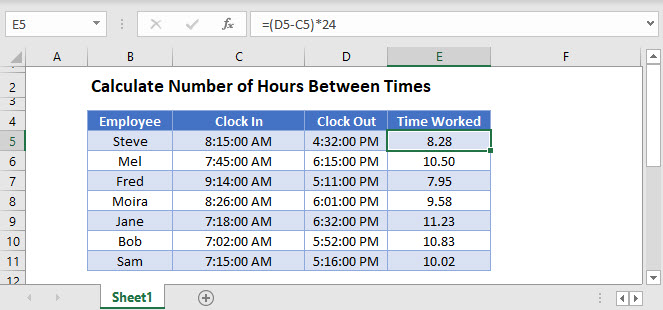## Calculate Hours with a Simple Formula

We can calculate the hours worked by deducting the end time from the start time.   The information has to be entered into Excel in a correct time format for the formula to work.

``=(D3-C3)*24``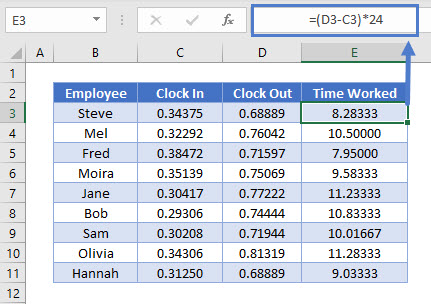Dates and Times are stored as numbers in Excel.   If we were to change the format of the start and end time columns in the above graphic to a general number format, we would get the numeric equivalents of the times shown.

Subtracting one column from the other gives us the difference between the two dates.  This will give us the percentage of the day worked based on 24 hours in a day.  To get the hours worked, we need to multiply this value by 24.

To format the values as time, select Home > Number >Time from the Ribbon.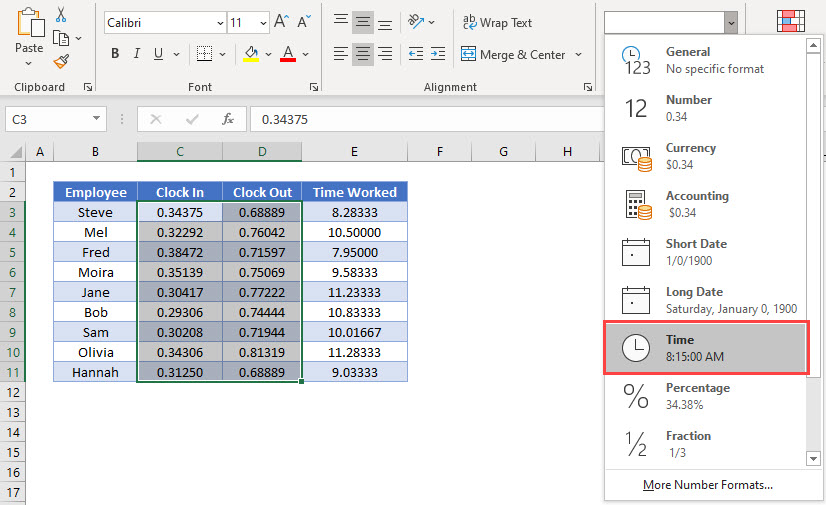To round the numbers for the Time worked to a single decimal place, we can use the ROUND function.

``=ROUND((D3-C3)*24,1)``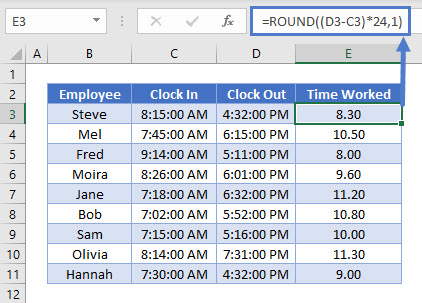## MROUND Function

When an employee clocks into a system, the system will record the exact time that that employee clocks in.  To get the Time clocked in and out to the nearest 15 minutes, we can use the MROUND function.

``=MROUND(C7, "0.15")``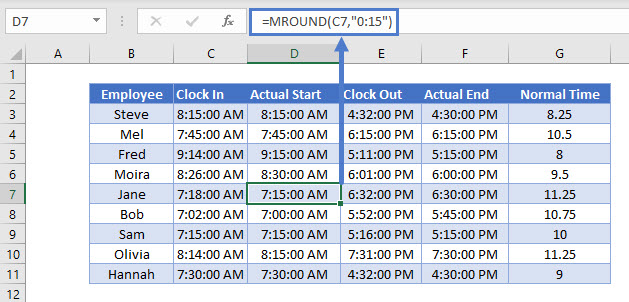If the clock in time is 7.18 am, then the MROUND function will round the time to the nearest 15 minutes ie to 7.15am.

We can then calculate the time worked using the Actual Start and Actual End times as shown in the example above.

If you want to round UP to the NEXT 15 minutes, you would use the CEILING Function.

``=CEILING(E3,"0.15")``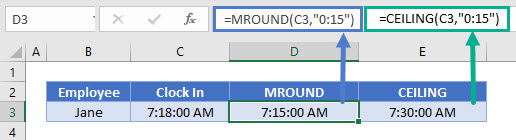Similarly, if you wish to round DOWN to the PREVIOUS 15 minutes, you would use the FLOOR Function.

``=FLOOR(E3, "0.15")``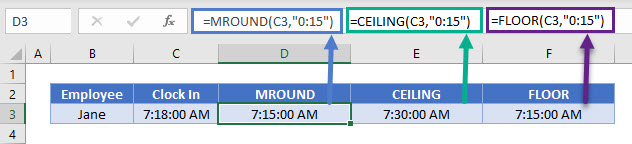## MOD Function

If the end time is before the start time, this may be due to a night shift being worked.  To solve the problem of the formula returning a negative number, we use the MOD function.

``=MOD(D3-C3,1)*24``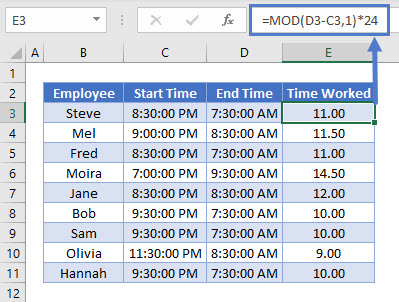As with the simple formula, we need to multiply the value calculated by the MOD function by 24 to get the hours worked.

## Calculating Hours Worked in Google Sheets

All the above Excel examples work the same way in Google Sheets.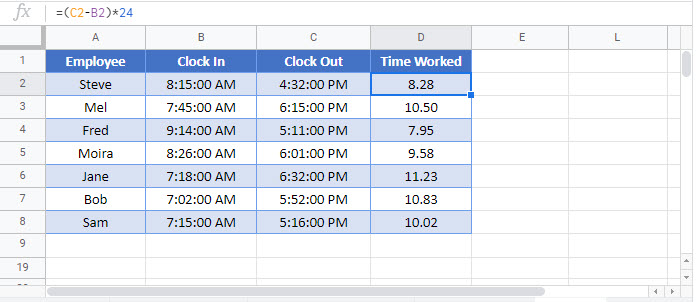### Excel Practice Worksheet

Practice Excel functions and formulas with our 100% free practice worksheets!